# Checker Detection/Localization

In this article I’ll discuss multiple ways to localize a checker in an image.

#### “opencv” method

The opencv method is the defacto standard for checker localization. It’s fast, robust, accurate and is the checker localization algorithm used in Bouguet’s camera calibration toolbox. It is based on the observation that a vector with its tail at the center of a checker and the tip in a region around a checker should always have a zero dot product with the intensity gradient located at the tip of the vector:

Note that the example figures in this section are for a corner, but the same holds for a checker. Anyway:

• in “flat” regions:• in edge regions:The dot product is zero in “flat” regions because the image gradient is zero there. In edge regions, it’s zero because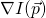and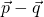are orthogonal:

You can use this constraint to set up a linear set of equations to solve forby sampling points (usually at integer pixel locations) around an initial guess of:

Here’s the math: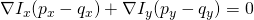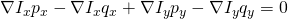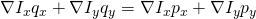Reformed as matrices: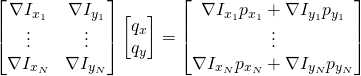So nowcan be solved for using a linear solver.

Some things you can do to improve this algorithm:

• Apply a Gaussian kernel to the sampling points aroundto give less weight to points further away from the checker center.
• Apply multiple iterations until convergence is reached (i.e. the position ofmoves less than a certain threshold)

If you’d like to see an example in action, you can download my camera calibration toolbox. The function which implements it is alg.refine_checker_opencv() and an example can be found in the unit tests folder.

#### “Edges” method

This is a cool method discussed in Mallon07. It tries to model the gradient magnitude of a checker with the following equation (note that I think the publication mistakenly switched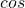and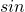in the first term):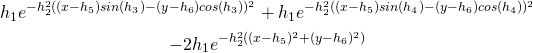This equation is actually pretty cool. The first two terms are essentially Gaussian distributions along lines, added together. Where the lines intersect, the Gaussian’s are added twice, and because the gradient magnitude is zero in that region for a checker, these values must be subtracted, hence the “-2” coefficient on the third term. The “h” coefficients are described below:

•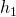– magnitude of the gradient peak
•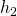– variance (i.e. width) of the gaussian distribution
•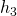– angle of first line
•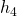– angle of second line
•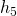– x component of line-line intersection
•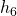– y component of line-line intersection

Since this equation is differentiable with respect to the “h” parameters, you can apply non-linear optimization to optimize them, and then get the center point from (,). I personally used matlab’s symbolic toolbox to do the symbolic differentiation since it helps prevent mistakes and is easier. I implemented the optimization with a simple Gauss Newton optimizer. One downside to this approach is you need to obtain initial guesses for the lines (even more difficult than an initial guess of a point needed for the opencv method). I did this by using a hough transform of the sub array containing the checker.

Below is an example of the optimization process:

Note that the final optimized edge function actually matches the array gradient for a checker very well.

If you’d like to see an example in action, you can download my camera calibration toolbox. The functions which implement it are alg.dominant_grad_angles() and alg.refine_checker_edges() and an example can be found in the unit tests folder.